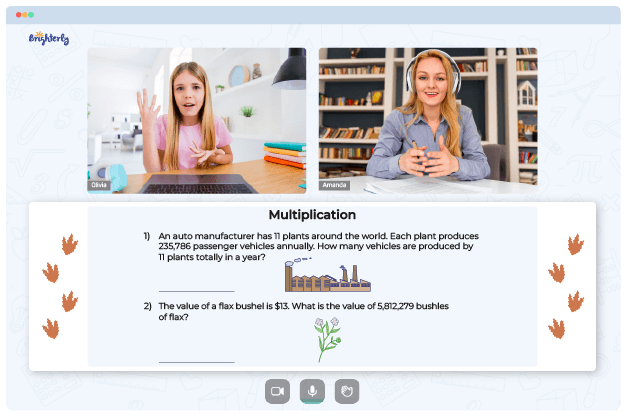# Completing The Square Worksheets

When you introduce the completing the squares method of solving quadratic equations to your students for the first time, you won’t need super perception to notice the confusion on their faces. While this approach to solving mathematical problems is straightforward, kids may find it complex for several reasons.

The chief of these reasons is the approach of the tutor. Hence, tutors and parents need a practical resource like a completing the square worksheet to help kids grasp this mathematical concept.

## Free completing the square practice worksheet from Brighterly

Solving quadratic equations by completing the square worksheets is one of the easiest ways your kids can learn. Completing the square methods of solving quadratic equations for grade school kids could be too challenging. Hence, having a math resource to assist them is a necessity.

Solving quadratic equations by completing the square worksheet should be easy for your kids with the numerous resources available on Brighterly. These worksheets, like all of Brighterly’s learning resources, are prepared by expert tutors with years of experience. The tutors do not just know about completing the squares; they are grounded in making kids learn with excitement.

Math for Kids

Is Your Child Struggling With Math?
1:1 Online Math TutoringAs a parent, you can rest assured that if your kids solve by completing the square worksheet from Brighterly, in no time, their excitement and interest in mathematics will be heightened. This math worksheet from Brighterly will boost your kids’ essential skills like critical thinking that they will need in their everyday life.

Since the worksheets comprise real-life graphics and attention-grabbing structure and color, your kids will be glued to them; therefore, they will learn more. As kids solve math problems in the worksheets continuously, it will heighten their self-confidence and belief in their ability.

Furthermore, the worksheets are deliberately organized in a “simplest to the most complex order.” Therefore, you don’t need to be scared that your kids will be overwhelmed with the worksheet questions. Your students will start from the foundation of the concept and proceed to the mid-level questions before encountering complex problems. This stepwise arrangement of tasks is necessary for kids to maintain interest in learning.

Solving quadratic equations by completing the square worksheet answer key questions will aid retention since they are arranged effectively. This structure will also boost kids’ problem-solving skills, especially as they try to answer complex questions after overcoming straightforward ones.### Completing The Square Worksheets PDF

Completing The Square Worksheet### Completing The Square Worksheets PDF

Solving Quadratic Equations By Completing The Square Worksheets### Completing The Square Worksheets PDF

Solving Quadratic Equations By Completing The Square Worksheet### Completing The Square Worksheets PDF

Completing The Square Practice Worksheet

## Printable completing the square worksheet algebra 2

You don’t have to sit in front of the computer whenever your kids want to learn while they complete the square worksheet. Make learning accessible to your kids and reduce distractions from using the computer by downloading the printable version of the worksheet. Learning continuously with these paper resources, your kids will become experts in less time.

Struggling with Multiplication?• Does your child struggle to master multiplication?
• Try studying with an online tutor.

Is your child having trouble understanding multiplication concepts? An online tutor could provide the necessary guidance.